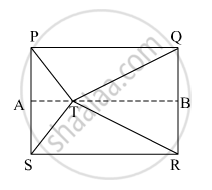Share

# In the Given Figure, Point T is in the Interior of Rectangle Pqrs, Prove That, Ts2 + Tq2 = Tp2 + Tr2 (As Shown in the Figure, Draw Seg Ab || Side Sr and A-t-b) - Geometry

ConceptPythagoras Theorem

#### Question

In the given figure, point T is in the interior of rectangle PQRS, Prove that, TS+ TQ= TP+ TR(As shown in the figure, draw seg AB || side SR and A-T-B)#### Solution

According to Pythagoras theorem, in ∆PAT

${PT}^2 = {PA}^2 + {AT}^2 . . . \left( 1 \right)$

In ∆ATS

${TS}^2 = {AT}^2 + {AS}^2 . . . \left( 2 \right)$

In ∆QBT

${QT}^2 = {QB}^2 + {BT}^2 . . . \left( 3 \right)$

In ∆BRT

${TR}^2 = {BT}^2 + {BR}^2 . . . \left( 4 \right)$

Now,

${TS}^2 + {TQ}^2 = \left( {AS}^2 + {AT}^2 \right) + \left( {QB}^2 + {BT}^2 \right) \left( \text{From} \left( 2 \right) \text{and} \left( 3 \right) \right)$

$\Rightarrow {TS}^2 + {TQ}^2 = \left( {BR}^2 + {AT}^2 \right) + \left( {PA}^2 + {BT}^2 \right) \left( \because AS = BR \text{ and } PA = QB \right)$

$\Rightarrow {TS}^2 + {TQ}^2 = \left( {BR}^2 + {BT}^2 \right) + \left( {PA}^2 + {AT}^2 \right)$

$\Rightarrow {TS}^2 + {TQ}^2 = \left( {TR}^2 \right) + \left( {PT}^2 \right) \left( \text{From} \left( 1 \right) \text{and} \left( 4 \right) \right)$

$\Rightarrow {TS}^2 + {TQ}^2 = {TR}^2 + {PT}^2$

Hence, TS+ TQ= TP+ TR2.

Is there an error in this question or solution?

#### Video TutorialsVIEW ALL 

Solution In the Given Figure, Point T is in the Interior of Rectangle Pqrs, Prove That, Ts2 + Tq2 = Tp2 + Tr2 (As Shown in the Figure, Draw Seg Ab || Side Sr and A-t-b) Concept: Pythagoras Theorem.
S